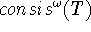## The incompleteness theorems

Gödel's first incompleteness theorem shows that any consistent logical theory expressive enough for elementary arithmetic, i.e. with addition, multiplication and quantifiers could express true sentences unprovable in the theory.

Gödel's second incompleteness theorem tells that the consistency of the system is one of these unprovable sentences.

The basis of Gödel's proof was the fact that the syntactic computations involved in combining formulas and verifying that a sequence of formulas is a proof can be imitated by arithmetic computations on ``Gödel numbers'' of formulas. If we have axioms for symbolic computations, e.g. for Lisp computations, then the proofs of Gödel's theorems become much shorter. Shankar [Shankar, 1986] has demonstrated this using the Boyer-Moore prover. Among the unprovable true sentences is the statement of the theory's own consistency. We can interpret this as saying that the theory lacks self-confidence. Turing, in his PhD thesis, studied what happens if we add to a theory T the statement consis(T) asserting that T is consistent, getting a stronger theory T'. While the new theory has consis(T) as a theorem, it doesn't have consis(T') as a theorem--provided it is consistent. The process can be iterated, and the union of all these theories is. Indeed the process can again be iterated, as Turing showed, to any constructive ordinal number.

John McCarthy
Mon Jul 15 13:06:22 PDT 2002